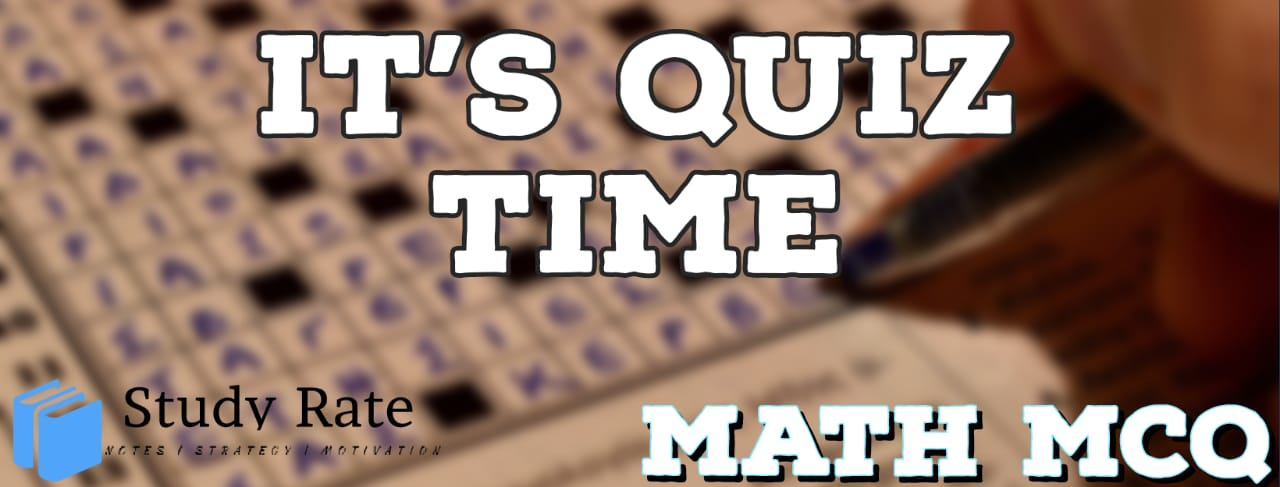## CBSE Class 10 Maths Chapter 7 –Coordinate Geometry MCQ’s Quiz

Free PDF Download of CBSE Class 10 Maths Chapter 7 –Coordinate Geometry Multiple Choice Questions with Answers. MCQ Questions for Class 10 Maths with Answers was Prepared Based on Latest Exam Pattern. Students can solve Class 10 Maths Coordinate Geometry MCQs with Answers to know their preparation level

Coordinate Geometry Class 10 Maths MCQ Online Quiz

1. What will be the reflection of the point (4, 5) about the X-axis, in the fourth quadrant?

2. Point P lies on the line 3x+ 4y – 12 = 0. If X- coordinate of P is a, then its y-coordinate is ______.

3.  If the distance between the points (2, –2) and (–1, x) is 5, one of the values of x is

4.  The mid-point of the line segment joining the points A (–2, 8) and B (– 6, – 4) is

5. A line intersects the y-axis and x-axis at the points P and Q, respectively. If (2, –5) is the mid – point of PQ, then the coordinates of P and Q are, respectively.
(A) (0, – 5) and (2, 0)      (B) (0, 10) and (– 4, 0)
(C) (0, 4) and (– 10, 0)    (D) (0, – 10) and (4, 0)

6. The line x + y = 10 divides line segment AB in the ratio a: 1. Find the value of a.7. If 2 triangles have the same height, the ratio of their areas is equal to the

8. The ratio in which the point P(3/4,5/12)  divides the line segment joining the Points A(1/2,3/2) and B (2, –5) is:

9. One of the two points of trisection of the line segment joining the points A (7, – 2) and B (1, – 5) which divides the line in the ratio 1:2 are:

10. The coordinates of the point which is equidistant from the three vertices of the Δ AOB as shown in the figure is: